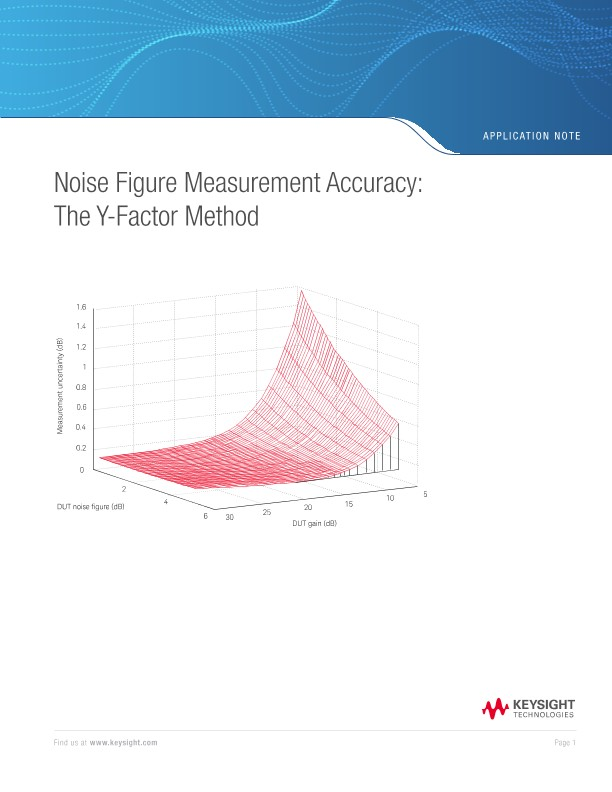# Noise Figure Measurement Accuracy: The Y-Factor Method

애플리케이션 노트

1 Introduction

Why is noise figure important?

Noise figure is a key performance parameter in many RF systems. A low noise figure provides an improved signal/noise ratio for analog receivers and reduces bit error rate in digital receivers. As a parameter in a communications link budget, a lower receiver noise figure allows smaller antennas or lower transmitter power for the same system performance.

In a development laboratory, noise figure measurements are essential to verify new designs and support existing equipment.

In a production environment, low-noise receivers can now be manufactured with minimal need for adjustment. Even so, it is still necessary to measure noise figures to demonstrate that the product meets specifications.

Why is accuracy important?

Accurate noise figure measurements have significant financial benefits. For many products, a guaranteed low noise figure commands a premium price. This income can only be realized, however, if every unit manufactured can be shown to meet its specification.

Every measurement has limits of accuracy. If a premium product has a maximum specified noise figure of 2.0 dB, and the measurement accuracy is ± 0.5 dB, then only units that measure 1.5 dB or lower are marketable. On the other hand, if the accuracy is improved to ± 0.2 dB, all products measuring up to 1.8 dB could be sold at the premium price.

Customers need accurate noise figure measurements to confirm they are getting the performance they have paid for. Using the same example, an accuracy of ± 0.5 dB for measuring a product that a manufacturer has specified as ‘2.0 dB maximum’ would require the acceptance of units measuring as high as 2.5 dB. An improved accuracy of ± 0.2 dB sets the acceptance limit at 2.2 dB.

Speed of measurement is also an issue. High-value products favor accuracy; highvolume products favor speed. Due to the random nature of noise and the statistical aspects of measuring it, there is always a trade-off between speed and accuracy.

To optimize the trade-off, it is necessary to eliminate all avoidable errors and quantify the uncertainties that remain.

This application note demonstrates how to improve noise figure measurement accuracy by following a three-stage process:

1. Avoid mistakes when making measurements
2. Minimize uncertainties wherever that is possible
3. Quantify the uncertainties that remain

This application note covers the following topics:

• Fundamentals of noise figure measurement using the Y-factor method. (Chapter 2)
• Noise figure mistakes to avoid (Chapter 3)
• Measurement corrections to improve accuracy (Chapter 4)
• Calculation of the remaining uncertainties – including software tools (Chapter 5)
• Other techniques that can reduce uncertainties (Chapter 6)
• Checklist for improving accuracy (Chapter 7).

This application note is specific to instruments that use the Y-factor method for noise figure measurement. Various features of Keysight Technologies products are mentioned as illustrative examples of the newest generation of noise figure analyzers and noise sources. Other products, however, may be used with the techniques discussed in this document.

2 Noise Figure Measurement

This chapter outlines the fundamental features of the Y-factor measurement technique for noise figure. Many instruments use the Y-factor technique, including:

• Keysight X-Series NFA noise figure analyzers (Figure 1-1)
• Keysight X-Series signal analyzers with noise figure measurement application
• Keysight FieldFox handheld microwave analyzers with noise figure measurements (Figure 1-2)
• Other noise figure analyzers and spectrum analyzers with noise figure measurement personality

The equations developed in this chapter follow the internal calculation route of the Keysight Technologies, Inc. products. The calculation routes of other noise figure instruments that use the Y-factor method are inevitably similar.

This chapter departs from previous explanations of noise figure calculations by making extensive use of the noise temperature concept. Although noise temperature may be less familiar, it gives a truer picture of how the instruments actually work—and most importantly, how they apply corrections to improve accuracy.

2.1 Fundamentals

2.1.1 What is noise figure?

As explained in Keysight’s Fundamentals of RF and Microwave Noise Figure Measurements, the fundamental definition of noise figure F is the ratio of:

(signal/noise power ratio at the input of the device under test)

(signal/noise power ratio at the output of the device under test)

Or alternatively:

Noise figure represents the degradation in signal/noise ratio as the signal passes through a device. Since all devices add a finite amount of noise to the signal, F is always greater than 1. Although the quantity F in equation 2-1 has historically been called ‘noise figure’, that name is now more commonly reserved for the quantity NF, expressed in dB:

Keysight Technologies literature follows the contemporary convention that refers to the ratio F as ‘noise factor’, and uses ‘noise figure’ to refer only to the decibel quantity NF.

×

판매 문의 부탁드립니다.

선호하는 연락방법을 선택해 주십시오. *필수입력항목
이메일 변경하기

체크 박스를 클릭하시면 이메일, 전화 및 문자 광고성 정보 수신동의를 의미합니다. 참고 : 이미 수신 동의를 한 경우 광고성 정보를 계속 받을 수 있으며, 만약 수신 거부를 원하는 경우 https://www.keysight.com/key/privacy에서 신청할 수 있습니다.
[키사이트 개인정보 수집 및 이용]

1. 개인정보 수집 및 이용 동의
2. 키사이트 파트너 업무 위탁 동의
3. 키사이트 해외 본사 및 지사 제공 동의
양식을 작성하여 제출하시면 개인정보 수집 및 이용에 동의한 것으로 간주합니다. 보다 자세한 내용은 개인정보보호정책을 참조하시기 바랍니다.

감사합니다!

A sales representative will contact you soon.